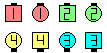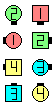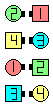Definitions of Square Dance Calls and Concepts

Ends \$B\$,GXCf9g\$o\$;\$NJ?9T\$J(B Line, \$B\$^\$?\$OB>\$NE,@Z\$J(B formation \$B\$+\$i!%(B

Ends \$B\$O(B Cross Fold and Roll \$B\$r\$7(B, Centers \$B\$O(B (Concentric \$B\$K(B) 1/4 Out and Trade \$B\$r\$7\$^\$9!%(B

\$BJ?9T\$J(B Line \$B\$+\$i\$O(B, \$B8~\$+\$\$9g\$C\$?(B Line \$B\$G=*\$o\$j\$^\$9!%(B2 \$B%Q!<%H\$N%3!<%k\$G\$9!%(BTrip The Set\$B\$NA0(B Ends Cross Fold as Centers 1/4 Out\$B\$N8e(B(1/2) Ends Roll as Centers Trade\$B\$N8e(B (\$B=*\$o\$j(B)